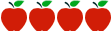### Grade 6: Expressions and Equations

Rating:http://hcpssfamilymath.weebly.com/expressions--equations-6ee7ee.html

#### Common Core Standards

Content Standards

6.EE.A.1 - Write and evaluate numerical expressions involving whole-number exponents.

6.EE.A.2 - Write, read, and evaluate expressions in which letters stand for numbers.

6.EE.A.3 - Apply the properties of operations to generate equivalent expressions.

6.EE.A.4 - Identify when two expressions are equivalent (i.e., when the two expressions name the same number regardless of which value is substituted into them).

6.EE.B.5 - Understand solving an equation or inequality as a process of answering a question: which values from a specified set, if any, make the equation or inequality true? Use substitution to determine whether a given number in a specified set makes an equation or inequality true.

6.EE.B.6 - Use variables to represent numbers and write expressions when solving a real-world or mathematical problem; understand that a variable can represent an unknown number, or, depending on the purpose at hand, any number in a specified set.

6.EE.B.7 - Solve real-world and mathematical problems by writing and solving equations of the form x + p = q andpx = q for cases in which pq and x are all nonnegative rational numbers.

6.EE.B.8 - Write an inequality of the form x > c or x < c to represent a constraint or condition in a real-world or mathematical problem. Recognize that inequalities of the form x > c orx < c have infinitely many solutions; represent solutions of such inequalities on number line diagrams.

6.EE.C.9 - Use variables to represent two quantities in a real-world problem that change in relationship to one another; write an equation to express one quantity, thought of as the dependent variable, in terms of the other quantity, thought of as the independent variable. Analyze the relationship between the dependent and independent variables using graphs and tables, and relate these to the equation.

Standards for Mathematical Practice

MP.1  Make sense of problems and persevere in solving them.

MP.2  Reason abstractly and quantitatively.

MP.3  Construct viable arguments and critique the reasoning of others.

MP.4  Model with mathematics.

MP.5  Use appropriate tools strategically.

MP.6  Attend to precision.

MP.7  Look for and make use of structure.

MP.8  Look for and express regularity in repeated reasoning.

#### Description of Unit

In this unit “Expressions and Equations” from the Howard County Public School System, projected to be 45 instructional days, students explore the use of variables in expressions.  They write expressions and equations that correspond to given situations, evaluate expressions, and use expressions and formulas to solve problems.  In some lessons, students will use the properties of operations to rewrite expressions in equivalent forms.  Students learn that the solutions of an equation are the values of the variables that make the equation true, and students will persevere in solving one step, two step, and multistep equations. Students construct and analyze tables, and they use equations to describe relationships between quantities. This is one of five units provided by Howard County Schools. Although Howard County is in a PARCC state, the assessment criteria still aligns with SBAC.

#### Cautions

Connecticut teachers should be aware that many lessons in this unit contain Illustrative Mathematics Tasks, the summative tasks or assessments are password protected. Therefore, Connecticut teachers should plan to create summative tasks or assessments. Rubrics or samples of student work are not included. Only limited lesson support is provided for students working above/below grade level, those students who are identified as students with disabilities and for English language learners (ELLs). Only limited support is provided for transfer across academic content domains.

#### Rationale for Selection

• Unit provides lessons that support the rigor of the Common Core State Standards.
• Unit provides detailed teacher resources and materials, as well as additional instructional support.
• Lessons demonstrate a good balance of procedural fluency and conceptual understanding.
• Uses and encourages precise and accurate mathematics, academic language, terminology, and concrete or abstract representations